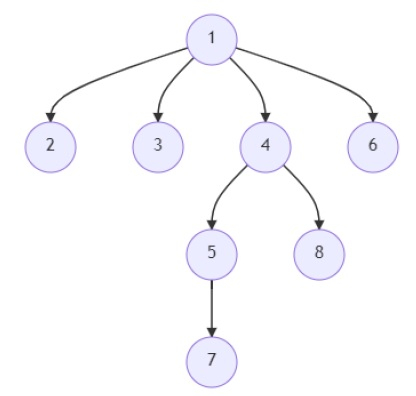# Program to find length of the longest path in an n-ary tree in Python

Suppose we have an edge list where each items are holding (u, v) represents u is parent of v. We have to find the length of the longest path in the tree. The path length is 1 + number of nodes in that path.

So, if the input is likethen the output will be 5, because the path is [1, 4, 5, 7], there are 4 nodes in total, so path length is 1 + 4 = 5.

To solve this, we will follow these steps −

• g := adjacency list of the graph from given edge list
• d := a new map
• Define a function bfs() . This will take o
• d[o] := 1
• f := o
• q := [o]
• for each x in q, do
• for each y in g[x], do
• if y is not in d, then
• d[y] := d[x] + 1
• if d[y] > d[f], then
• f := y
• insert y in q
• return f
• From the main method, do the following −
• for each o in g, do
• f := bfs(o)
• d := a new map
• return d[bfs(f)]
• return 0

## Example

Let us see the following implementation to get better understanding −

def solve(edges):
g = {}
for u, v in edges:
if u not in g:
g[u] = []
g[u] += (v,)
if v not in g:
g[v] = []
g[v] += (u,)
d = {}

def bfs(o):
d[o] = 1
f = o
q = [o]
for x in q:
for y in g[x]:
if y not in d:
d[y] = d[x] + 1
if d[y] > d[f]:
f = y
q += (y,)
return f

for o in g:
f = bfs(o)
d = {}
return d[bfs(f)]
return 0

edges = [(1, 2),(1, 3),(1, 4),(4, 5),(5,7),(1,6),(4,8)]
print(solve(edges))

## Input

[(1, 2),(1, 3),(1, 4),(4, 5),(5,7),(1,6),(4,8)]

## Output

5

Updated on: 19-Oct-2021

159 Views